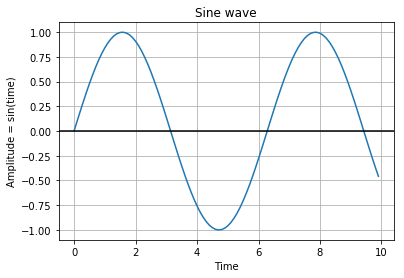Nikola Jupyter Notebook Publishing

This week I spent some time converting my Wordpress blog over to a static site generator; Nikola. If you were led here to figure out how to do this, then I suggest you go here:

https://mglerner.github.io/posts/switching-to-nikloa-for-jupyter-notebooks-and-a-static-site.html

In :
%matplotlib inline


Sample code to generate a sine wave

In :
import numpy as np
import matplotlib.pyplot as plt

time = np.arange(0, 10, 0.1);
amplitude   = np.sin(time)
plt.plot(time, amplitude)
plt.title('Sine wave')
plt.xlabel('Time')
plt.ylabel('Amplitude = sin(time)')
plt.grid(True, which='both')
plt.axhline(y=0, color='k')

plot.show()In [ ]: